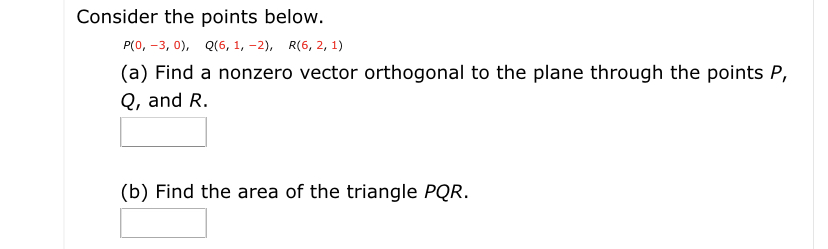# Consider the points below.P(0, -3, 0), Q(6, 1, -2), R(6, 2, 1)(a) Find a nonzero vector orthogonal to the plane through the points P,Q, and R.(b) Find the area of the triangle PQR.

Question
1 viewshelp_outlineImage TranscriptioncloseConsider the points below. P(0, -3, 0), Q(6, 1, -2), R(6, 2, 1) (a) Find a nonzero vector orthogonal to the plane through the points P, Q, and R. (b) Find the area of the triangle PQR. fullscreen
check_circle

star
star
star
star
star
1 Rating
Step 1

P(0,-3,0) , Q(6,1,-2), R(6,2,1)

Find two vectors on the plane PQ and PR

Step 2

Cross product of PQ and PR produces a vector orthogonal to the tw...

### Want to see the full answer?

See Solution

#### Want to see this answer and more?

Solutions are written by subject experts who are available 24/7. Questions are typically answered within 1 hour.*

See Solution
*Response times may vary by subject and question.
Tagged in

### Other